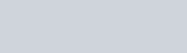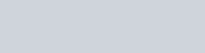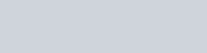Three electrolytic cells A,B,C containing solutions of
Question:

Three electrolytic cells A,B,C containing solutions of ZnSO4, AgNO3 and CuSO4, respectively are connected in series. A steady current of 1.5 amperes was passed through them until 1.45 g of silver deposited at the cathode of cell B. How long did the current flow? What mass of copper and zinc were deposited?

Solution:

According to the reaction:i.e., 108 g of Ag is deposited by 96487 C.

Therefore, $1.45 \mathrm{~g}$ of $\mathrm{Ag}$ is deposited by $=\frac{96487 \times 1.45}{108} \mathrm{C}$

= 1295.43 C

Given,

Current = 1.5 A

$\therefore$ Time $=\frac{1295.43}{1.5} \mathrm{~s}$

= 863.6 s

= 864 s

= 14.40 min

Again,i.e., 2 × 96487 C of charge deposit = 63.5 g of Cu

Therefore, $1295.43 \mathrm{C}$ of charge will deposit $=\frac{63.5 \times 1295.43}{2 \times 96487} \mathrm{~g}$

= 0.426 g of Cui.e., 2 × 96487 C of charge deposit = 65.4 g of Zn

Therefore, $1295.43 \mathrm{C}$ of charge will deposit $=\frac{65.4 \times 1295.43}{2 \times 96487} \mathrm{~g}$

= 0.439 g of Zn